Next: General Relation Between Specific Up: Classical Thermodynamics Previous: Helmholtz Free Energy

# Gibbs Free Energy

Suppose, finally, thatandare the are the two independent parameters that specify the system. Because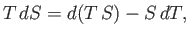(6.110)

we can rewrite Equation (6.91) in the form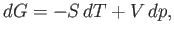(6.111)

where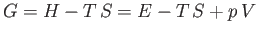(6.112)

is termed the Gibbs free energy.

Proceeding as before, we write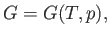(6.113)

which implies that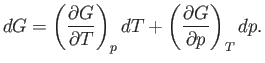(6.114)

Comparison of Equations (6.111) and (6.114) yields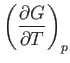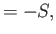(6.115)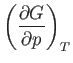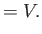(6.116)

We also know that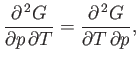(6.117)

or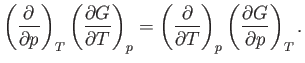(6.118)

Thus, it follows from Equations (6.115) and (6.116) that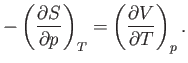(6.119)

Equations (6.89), (6.99), (6.109), and (6.119) are known collectively as Maxwell relations.Next: General Relation Between Specific Up: Classical Thermodynamics Previous: Helmholtz Free Energy
Richard Fitzpatrick 2016-01-25